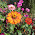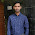## Saturday, September 8, 2012

### Electrical Machines: Part 3

The size of the feeder is determined primarily by
(a) The current it is required to carry.
(b) The percent variation of voltage in the feeder.
(c) The voltage across the feeder.
(d) The distance of transmission.

Answer: A

 The emf induced in the primary of a transformer
(a) Is in phase with the flux.
(b) Lags behind the flux by 90 degree.
(c) Leads the flux by 90 degree.
(d) Is in phase opposition to that of flux.

Answer: C

 The relative speed between the magnetic fields of stator and rotor under steady state operation is zero for a
(a) DC machine.
(b) 3 phase induction machine.
(c) Synchronous machine.
(d) Single phase induction machine.

Answer: all options are correct

 As the voltage of transmission increases, the volume of conductor
(a) Increases.
(b) Does not change.
(c) Decreases.
(d) Increases proportionately.

Answer: C

Due to skin effect
 In a 3-phase synchronous motor
(a) The speed of stator MMF is always more than that of rotor MMF.
(b) The speed of stator MMF is always less than that of rotor MMF.
(c) The speed of stator MMF is synchronous speed while that of rotor MMF is zero.
(d) Rotor and stator MMF are stationary with respect to each other.

Answer: D

Motor is magnetically locked into position with stator, the rotor poles are engaged with stator poles and both run synchronously in same direction.
 An alternator is delivering rated current at rated voltage and 0.8 power-factor lagging case. If it is required to deliver rated current at rated voltage and 0.8 power-factor leading, the required excitation will be
(a) Less.
(b) More.
(c) More or less.
(d) The same.

Answer: B

Over excitation gives leading power factor and under excitation gives lagging p.f .
 Out of the following methods of heating the one which is independent of supply frequency is
(a) Electric arc heating
(b) Induction heating
(c) Electric resistance heating
(d) Dielectric heating

Answer: C

 In a capacitor start single-phase induction motor, the capacitor is connected
(a) In series with main winding.
(b) In series with auxiliary winding.
(c) In series with both the windings.
(d) In parallel with auxiliary winding.

Answer: B

To make single phase motor self start. We split the phases at 90 degree. Hence, motor behaves like a two phase motor.
 A synchro has
(a) A 3-phase winding on rotor and a single-phase winding on stator.
(b) A 3-phase winding on stator and a commutator winding on rotor.
(c) A 3-phase winding on stator and a single-phase winding on rotor.
(d) A single-phase winding on stator and a commutator winding on rotor.

Answer: C

The basic synchro unit called a synchro transmitter. It’s construction similar to that of a Three phase alternator.
 A ceiling fan uses
(a) Split-phase motor.
(b) Capacitor start and capacitor run motor.
(c) Universal motor.
(d) Capacitor start motor.

Answer: D

To give starting torque and to maintain speed.
6:05 AM

#### 4 comments:

1.questions and answers r helpful undoubtedly but numerical problems should be answer with a little explanation or atleast the formula its using

2.question no 6 explanation is valid for sync motor....but just happens reverse in alternator as asked in question

3.4.Question No.6 is wrong ---> in alternator leading power factor gives under excitation whereas over excitation gives Lagging power factor so the answer is A